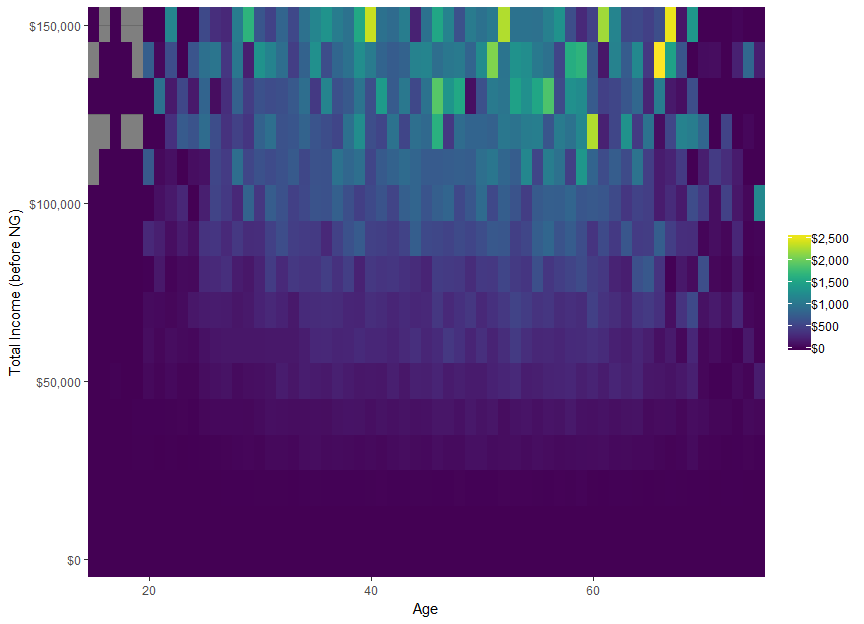# Companion to 2013-14 sample file

## Prologue

This vignette is a mirror of a small book prepared internally by Grattan Institute. The goal is to demonstrate how to perform simple analysis and create common charts. You will need the taxstats package available via devtools::install_github('hughparsonage/taxstats').

options("scipen" = 99)
library(knitr)
opts_chunk$set(fig.width = 9, fig.height = 6.5) FY.YEAR <- "2013-14" wsum <- function(x, w = 1){ sum((x) * w) } library(data.table) if (requireNamespace("taxstats", quietly = TRUE)){ library(taxstats) sample_files_all <- get_sample_files_all() } else { install.packages("taxstats", repos = "https://hughparsonage.github.io/drat/", type = "source") library(taxstats) sample_files_all <- get_sample_files_all() } library(grattan) library(dtplyr) library(dplyr) library(ggplot2) library(scales) library(magrittr) library(ggrepel) library(viridis) ## Loading required package: viridisLite ## ## Attaching package: 'viridis' ## The following object is masked from 'package:scales': ## ## viridis_pal if (!exists("sample_files_all")){ stop("....") } grattan_dollar <- function (x, digits = 0) { # nsmall <- digits commaz <- format(abs(x), nsmall = nsmall, trim = TRUE, big.mark = ",", scientific = FALSE, digits = 1L) if_else(x < 0, paste0("\U2212","$", commaz),
paste0("$", commaz)) } sample_file <- sample_files_all %>% filter(fy.year == FY.YEAR) sample_file <- merge(sample_file, age_range_decoder, by = "age_range") PREV.FY.YEAR <- yr2fy(fy2yr(FY.YEAR) - 1) sample_file_prev <- sample_files_all[fy.year == PREV.FY.YEAR] sample_file_prev <- merge(sample_file_prev, age_range_decoder, by = "age_range") set.seed(48031) sample_file %<>% group_by(age_range_description) %>% mutate(min_age = ifelse(grepl("to", age_range_description), as.numeric(gsub("^([0-9]{2}).*$", "\\1", age_range_description)),
ifelse(grepl("70", age_range_description),
70,
15)),
max_age = min_age + 5,
age_imp = runif(n(), min_age, max_age)) %>%
select(-min_age, -max_age)
sample_file %<>%
mutate(Tax_Bracket = cut(Taxable_Income,
breaks = c(-Inf, 18200, 37e3, 80e3, 180e3, Inf),
include.lowest = TRUE,
labels = c("$0-$18,200",
"$18,201-$37,000",
"37,001-$80,000", "$80,001-$180,000", "$180,000+")))
texNum <- function(number, sig.figs = 3L, dollar = FALSE, pre.phrase = NULL, .suffix = NULL){
orig.number <- number
stopifnot(is.numeric(number), length(number) == 1L)
is.negative <- number < 0
number <- abs(number)
if (number == 0){
warning("Returning 0")
return(0)
} else {
if (is.null(.suffix)){
n.digits <- ceiling(log10(number))

suffix <- NULL
suffix_val <- 1

if (n.digits < sig.figs){
prefix <- signif(x = number, digits = sig.figs)
} else {

if (n.digits <= 6) {
prefix_val <- round(number, sig.figs - n.digits - 1)
prefix <- prettyNum(prefix_val, big.mark = ",", scientific = FALSE)
} else {
# Want to show only the number / 10^(multiple of 3) then the suffix multiplier
suffix_val <- 10 ^ (3 * ((n.digits %/% 3)))
prefix_val <- signif(number/suffix_val, digits = sig.figs)
prefix <- prefix_val

if (suffix_val <= 10^12){
switch(log10(suffix_val) / 3 - 1,
suffix <- "~million",
suffix <- "~billion",
suffix <- "~trillion")
} else {
prefix <- signif(number / 10^12, digits = sig.figs)
suffix <- "~trillion"
}
}
}
} else {
stopifnot(.suffix %in% c("million", "billion", "trillion"))
switch(.suffix,
"million" = {
prefix <- signif(number / 10^6, digits = sig.figs)
suffix <- "~million"
suffix_val <- 10^6
},
"billion" = {
prefix <- signif(number / 10^9, digits = sig.figs)
suffix <- "~billion"
suffix_val <- 10^9
},
"trillion" = {
prefix <- signif(number / 10^12, digits = sig.figs)
suffix <- "~trillion"
suffix_val <- 10^12
})
prefix_val <- prefix
}

if (dollar){
out <- paste0("\\$", prefix, suffix) } else { out <- paste0(prefix, suffix) } if (is.negative){ # General LaTeX out <- paste0("\$$-\$$", out) } # is the displayed number larger than the original? if (!is.null(pre.phrase)){ out_larger <- prefix_val * suffix_val > orig.number if (out_larger) { out <- paste(pre.phrase, out, sep = if(grepl("~$", pre.phrase)) "" else " ")
} else {
if (!isTRUE(all.equal(prefix_val * suffix_val,
orig.number,
tolerance = .Machine$double.eps))) out <- paste(pre.phrase, out, sep = if(grepl("~$", pre.phrase)) "" else " ")
}

}
return(out)
}
}

There were 12.9~million taxpayers in 2013-14 in Australia. Of those, 180,000 had zero taxable income (or a taxable loss). (… and so these ‘’taxpayers’’ naturally paid no tax. Nor did the 2.5~million individuals below the tax-free threshold. For this vignette, a taxpayer is anyone who lodged a tax return, regardless of their tax liability).

tx_inc_q <- function(q){
quantile(sample_file$Taxable_Income, probs = q) } my_labs <- grattan_dollar(tx_inc_q((0:10)/10)) my_labs[seq(2, 10, 2)] <- paste0("\n", my_labs[seq(2, 10, 2)]) dens <- density(sample_file[Taxable_Income < tx_inc_q(0.95)]$Taxable_Income)
DF <- with(dens, data.frame(x, y))

sample_file %>%
mutate(Taxable_Income_decile = ntile(Taxable_Income, 10)) %>%
filter(between(Taxable_Income, 0, tx_inc_q(0.95))) %>%
ggplot(aes(x = Taxable_Income)) +
geom_density() +
scale_fill_viridis(discrete = TRUE) +
scale_x_continuous("Taxable Income deciles",
labels = c(my_labs, grattan_dollar(tx_inc_q(0.95))),
# limits = c(0, tx_inc_q(0.95)),
breaks = c(tx_inc_q((0:10)/10), tx_inc_q(0.95))) +
scale_y_continuous(expand = c(0,0)) +
theme(legend.position = "none",
axis.line.y = element_blank(),
axis.text.y = element_blank(),
axis.title.y = element_blank())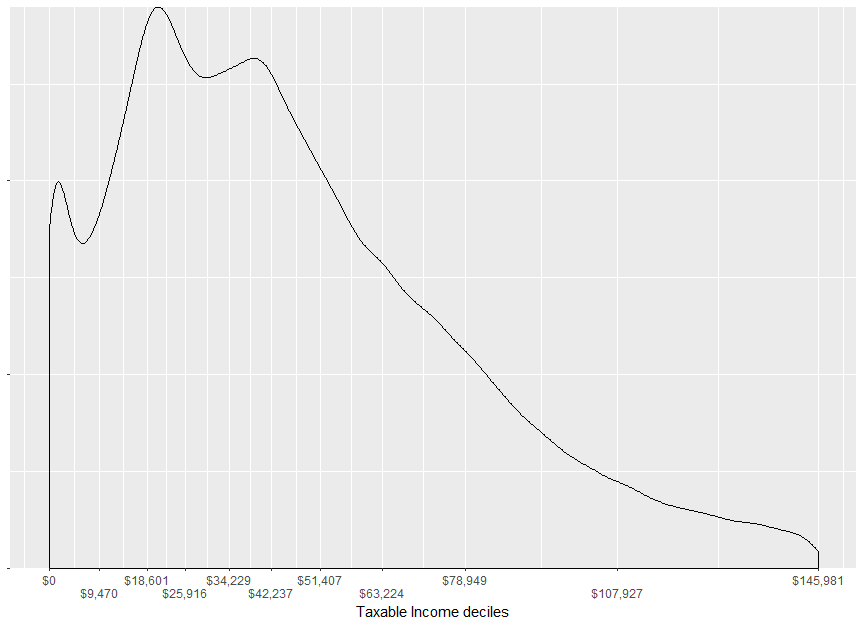DF %>%
mutate(Taxable_Income_decile = cut(x,
breaks = quantile(sample_file$Taxable_Income, probs = c(0:10)/10), right = TRUE, include.lowest = TRUE)) %>% filter(between(x, -1, tx_inc_q(0.95) * 1.05)) %>% { ggplot(., aes(x = x, y = y)) + geom_area(color = "black", size = 1.45) + geom_area(aes(x = x, y = y, group = Taxable_Income_decile, fill = factor(Taxable_Income_decile), color = factor(Taxable_Income_decile))) + scale_color_viridis(discrete = TRUE) + scale_fill_viridis(discrete = TRUE) + scale_x_continuous("Taxable Income deciles", labels = c(my_labs, grattan_dollar(tx_inc_q(0.95))), expand = c(0,0), # limits = c(-1, tx_inc_q(0.95)*1.05), breaks = c(tx_inc_q((0:10)/10), tx_inc_q(0.95))) + scale_y_continuous(expand = c(0,0), limits = c(0, max(.$y) * 1.05)) +
theme(legend.position = "none",
axis.line.y = element_blank(),
axis.text.y = element_blank(),
axis.title.y = element_blank())+

annotate("text",
x = tx_inc_q(0.925),
y = 2 * max(.$y[.$x > tx_inc_q(0.925)]),
size = 10/(14/5),
label = paste0("5% of taxpayers\nhad incomes\ngreater than\n", grattan_dollar(tx_inc_q(0.95))),
hjust = 0,
vjust = 0) +
annotate("segment",
arrow = arrow(type = "closed", length = unit(11, "pt"), angle = 20),
x = tx_inc_q(0.925),
y = 1.9 * max(.$y[.$x > tx_inc_q(0.925)]),
size = 1,
xend = tx_inc_q(0.95),
yend = 1.9 * max(.$y[.$x > tx_inc_q(0.925)]))
}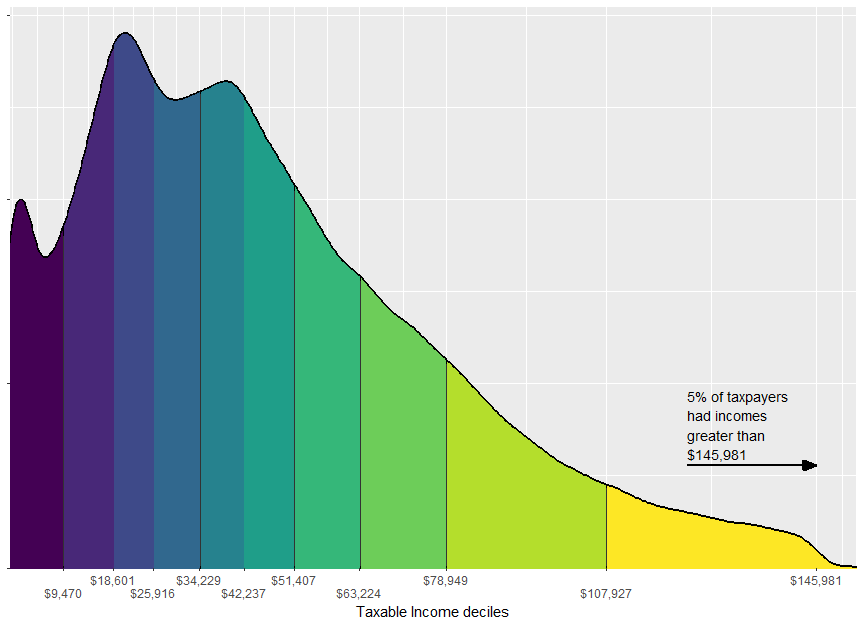n_CGs <-
sample_file %>%
filter(Tot_CY_CG_amt > 0) %$% sum(WEIGHT) n_CGs_prev <- sample_file_prev %>% filter(Tot_CY_CG_amt > 0) %$%
sum(WEIGHT)

tot_CG_amt <-
sample_file %$% sum(as.numeric(Tot_CY_CG_amt * WEIGHT)) tot_Net_CG_amt <- sample_file %$%
sum(as.numeric(Net_CG_amt * WEIGHT))

tax_on_CG <-
sample_file %>%
filter(Net_CG_amt > 0) %>%
mutate(tax = income_tax(Taxable_Income, fy.year = FY.YEAR),
tax_wo_CG = income_tax(pmaxC(Taxable_Income - Net_CG_amt, 0), fy.year = FY.YEAR)) %>%
summarise(total = sum((tax - tax_wo_CG) * WEIGHT),
avg = mean(tax - tax_wo_CG))

tax_on_CG_prev <-
sample_file_prev %>%
filter(Net_CG_amt > 0) %>%
mutate(tax = income_tax(Taxable_Income, fy.year = FY.YEAR),
tax_wo_CG = income_tax(pmaxC(Taxable_Income - Net_CG_amt, 0), fy.year = FY.YEAR)) %>%
summarise(total = sum((tax - tax_wo_CG) * WEIGHT),
avg = mean(tax - tax_wo_CG))
latex_percent <- function(x) gsub("%", "\\%", percent(x), fixed = TRUE)

The capital gains discount applies to assets sold after more than 12 months’ holding. There were 840,000 individuals who sold capital assets, up 8.84% from last year. The sale of their assets totalled $34.2~billion of which$12.9~billion comprised part of their taxable income.

The tax on these capital gains totalled $4.76~billion or$7,900 per individual with capital gains tax.

probCG_by_age <-
sample_file %>%
group_by(age_range_description) %>%
summarise(probCG = mean(Net_CG_amt > 0))

probCG_twenties <-
sample_file %>%
filter(age_imp < 30) %$% mean(Net_CG_amt > 0) probCG_65p <- sample_file %>% filter(age_imp >= 65) %$%
mean(Net_CG_amt > 0)

avg_marginal_rate_CG <-
sample_file %>%
filter(Net_CG_amt > 0) %>%
mutate(marginal_rate = income_tax(Taxable_Income + 1, fy.year = FY.YEAR) - income_tax(Taxable_Income, fy.year = FY.YEAR)) %$% mean(marginal_rate) avg_marginal_rate_CG_weighted_by_CG <- sample_file %>% filter(Net_CG_amt > 0) %>% mutate(marginal_rate = income_tax(Taxable_Income + 1, fy.year = FY.YEAR) - income_tax(Taxable_Income, fy.year = FY.YEAR)) %$%
weighted.mean(marginal_rate, Net_CG_amt)

avg_marginal_rate_b4_CG <-
sample_file %>%
filter(Net_CG_amt > 0,
age_imp >= 20) %>%
mutate(Taxable_Income_b4_CG = pmaxC(Taxable_Income - Net_CG_amt, 0),
marginal_rate_b4_CG = income_tax(Taxable_Income_b4_CG + 1, fy.year = FY.YEAR) - income_tax(Taxable_Income_b4_CG, fy.year = FY.YEAR)) %>%
mutate(is_in_workforce = between(age_imp, 20, 65)) %>%
group_by(is_in_workforce) %>%
summarise(avg_marginal_rate_weighted = weighted.mean(marginal_rate_b4_CG, Net_CG_amt),
avg_marginal_Rate = mean(marginal_rate_b4_CG))

prop_no_CGT_discount <-
sample_file %>%
mutate(apparent_discount = 1 - Net_CG_amt / Tot_CY_CG_amt) %>%
filter(Tot_CY_CG_amt > 0) %$% mean(apparent_discount == 0) prop_100pc_CGT_discount <- sample_file %>% mutate(apparent_discount = 1 - Net_CG_amt / Tot_CY_CG_amt) %>% filter(Tot_CY_CG_amt > 0) %$%
mean(apparent_discount == 1)

prop_50pc_CGT_discount <-
sample_file %>%
mutate(apparent_discount = 1 - Net_CG_amt / Tot_CY_CG_amt) %>%
filter(Tot_CY_CG_amt > 0) %$% mean(between(apparent_discount, 0.45, 0.55)) prop_no_CGT_discount_by_val <- sample_file %>% mutate(apparent_discount = 1 - Net_CG_amt / Tot_CY_CG_amt) %>% filter(Tot_CY_CG_amt > 0) %$%
weighted.mean(apparent_discount == 0, Tot_CY_CG_amt)

cgt_ratio_res <- 50
sample_file %>%
select(Tot_CY_CG_amt, Net_CG_amt, WEIGHT) %>%
filter(Tot_CY_CG_amt > 0) %>%
mutate(apparent_discount = Net_CG_amt / Tot_CY_CG_amt) %>%
mutate(apparent_discount_round = round(apparent_discount * cgt_ratio_res, 0) / cgt_ratio_res) %>%
group_by(apparent_discount_round) %>%
summarise(n_taxpayers = sum(WEIGHT),
n_taxpayers_by_val = sum(WEIGHT * Tot_CY_CG_amt)) %>%
rename(Ratio of Net capital gains to Total capital gains = apparent_discount_round) %>%
ggplot(aes(x = Ratio of Net capital gains to Total capital gains, y = n_taxpayers_by_val)) +
geom_bar(stat = "identity", width = 1/cgt_ratio_res) +
theme(axis.title.y = element_blank(),
axis.ticks.y = element_blank(),
axis.text.y = element_blank())
## Warning in sum(WEIGHT * Tot_CY_CG_amt): integer overflow - use
## sum(as.numeric(.))

## Warning in sum(WEIGHT * Tot_CY_CG_amt): integer overflow - use
## sum(as.numeric(.))
## Warning: Removed 2 rows containing missing values (position_stack).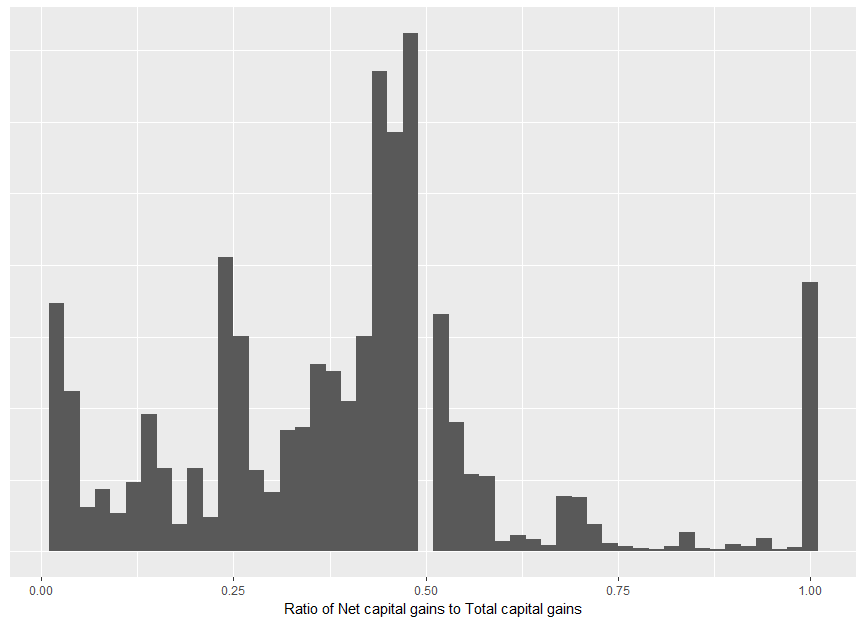Taxable capital gains are typically realized later in life. This is unsurprising: a capital gain can only be realized when one has an asset to sell. Further, the capital gains tax makes the sale of assets less attractive when incomes are high. Taxpayers in their twenties have a 1.28% chance of incurring capital gains tax, whereas 11.8% of those of retirement age have capital gains. shows that although capital gains have been more common with older taxpayers, the age skew is slightly more pronounced in 2013-14 than in previous years.

The average marginal tax rate of those with capital gains tax was 29.1%; however, this weights an individual with a capital gain of $1 equally as someone with a capital gain of$500,000. Weighting by the value of capital gain, the average marginal tax rate was 40.6%.

The net capital gains includes the CGT discount (and other discounts) applied to: $\text{Total capital gains} - \text{Total capital losses (incl. from prev. years)}$ Comparing the ratio of can shed some light on the value of the discount and the impact of capital losses on tax and tax revenue. Of those with nonzero total capital gains, 8.79% had no discount and 28.5% paid no tax (or a 100% discount). Some 45.3% had net capital gains of around 50% of their total gains. Weighting these numbers by the value of total capital gains, of capital gains are taxed at the full marginal rate. shows the distribution of this ratio. The deviance from 50% is due to some gains being realized within 12 months and (more commonly) capital losses.

CG_descriptive_by_bracket <-
sample_file %>%
mutate(tax = income_tax(Taxable_Income, fy.year = FY.YEAR),
tax_wo_CG = income_tax(pmaxC(Taxable_Income - Net_CG_amt, 0), fy.year = FY.YEAR)) %>%
group_by(Tax_Bracket) %>%
summarise(n_taxpayers = sum(WEIGHT),
n_CG = sum(WEIGHT[Net_CG_amt > 0]),
val_CG = sum(Tot_CY_CG_amt * WEIGHT),
total_CGT = sum((tax - tax_wo_CG) * WEIGHT)) %>%
ungroup %>%
arrange(Tax_Bracket) 
## Warning in sum(Tot_CY_CG_amt * WEIGHT): integer overflow - use
## sum(as.numeric(.))

## Warning in sum(Tot_CY_CG_amt * WEIGHT): integer overflow - use
## sum(as.numeric(.))

## Warning in sum(Tot_CY_CG_amt * WEIGHT): integer overflow - use
## sum(as.numeric(.))

## Warning in sum(Tot_CY_CG_amt * WEIGHT): integer overflow - use
## sum(as.numeric(.))
CG_descriptive_by_bracket %>%
# cosmetic
mutate(Taxpayers = comma(n_taxpayers),
with CG = comma(n_CG),
Total cap. gains ($) = grattan_dollar(val_CG), Total CGT ($) = grattan_dollar(total_CGT)) %>%
select(Tax bracket = Tax_Bracket,
Taxpayers, with CG, Total cap. gains ($), Total CGT ($)) %>%
kable(align = "rrrrrr") 
Tax bracket Taxpayers with CG Total cap. gains ($) Total CGT ($)
$0-$18,200 2,512,650 84,000 NA $0$18,201-$37,000 3,101,900 118,300$1,958,287,950 $64,130,858 37,001-$80,000 4,808,650 185,600 NA $413,550,412$80,001-$180,000 2,135,400 151,350 NA$965,377,002
$180,000+ 380,100 61,150 NA$3,314,621,140
sample_file %>%
ggplot(aes(x = age_imp, y = as.numeric(Net_CG_amt > 0))) +
geom_smooth(color = viridis(1), size = 1.2) +
scale_y_continuous(label = percent) 
## geom_smooth() using method = 'gam' and formula 'y ~ s(x, bs = "cs")'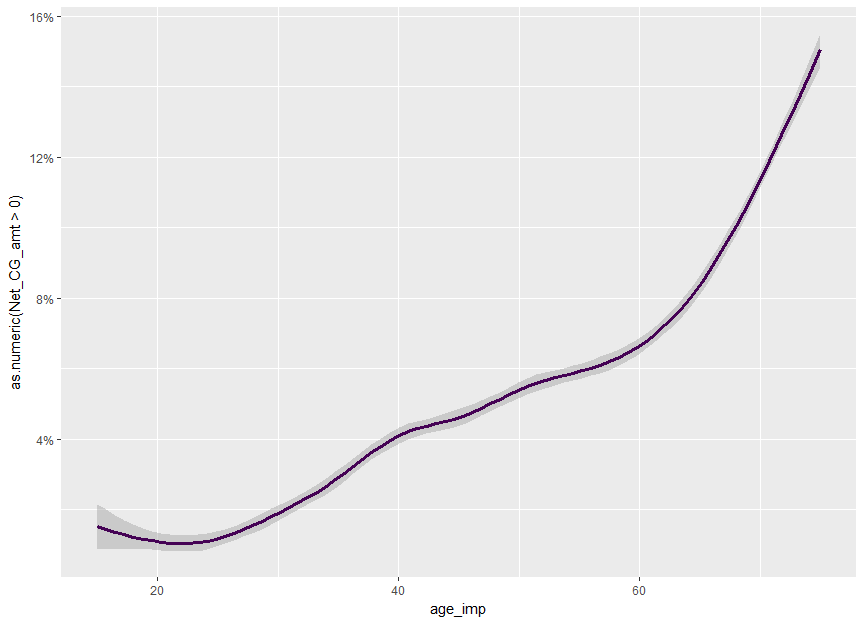sample_files_all %>%
select(age_range, Net_CG_amt, fy.year) %>%
merge(age_range_decoder, by = "age_range") %>%
group_by(age_range_description) %>%
mutate(min_age = ifelse(grepl("to", age_range_description),
as.numeric(gsub("^([0-9]{2}).*$", "\\1", age_range_description)), ifelse(grepl("70", age_range_description), 70, 15)), max_age = min_age + 5, age_imp = runif(n(), min_age, max_age)) %>% select(-min_age, -max_age) %>% mutate(last_fy = fy.year == max(fy.year)) %>% mutate(Tax year = factor(fy.year)) %>% group_by(Tax year) %>% mutate(label = if_else(age_imp == max(age_imp), fy.year, NA_character_), is_CG = Net_CG_amt > 0, label.y = mean(is_CG[age_imp > 71]), Age = age_imp) %>% { ggplot(., aes(x = Age, y = as.numeric(is_CG), color = Tax year, group = Tax year)) + scale_y_continuous(label = percent) + ggtitle("Incidence of capital gains") + scale_color_viridis(discrete = TRUE) + geom_line(stat = "smooth", method = "auto", se = FALSE, size = 1.2) + geom_label_repel(aes(label = label, y = label.y), fill = NA, nudge_x = 1, hjust = 0, vjust = 0, fontface = "bold", na.rm = TRUE) + annotate("blank", x = 80, y = 0) + theme_dark() + theme(axis.title.y = element_blank()) } ## Warning: Ignoring unknown parameters: hjust, vjust ## Warning: Ignoring unknown aesthetics: x, y ## geom_smooth() using method = 'gam' and formula 'y ~ s(x, bs = "cs")'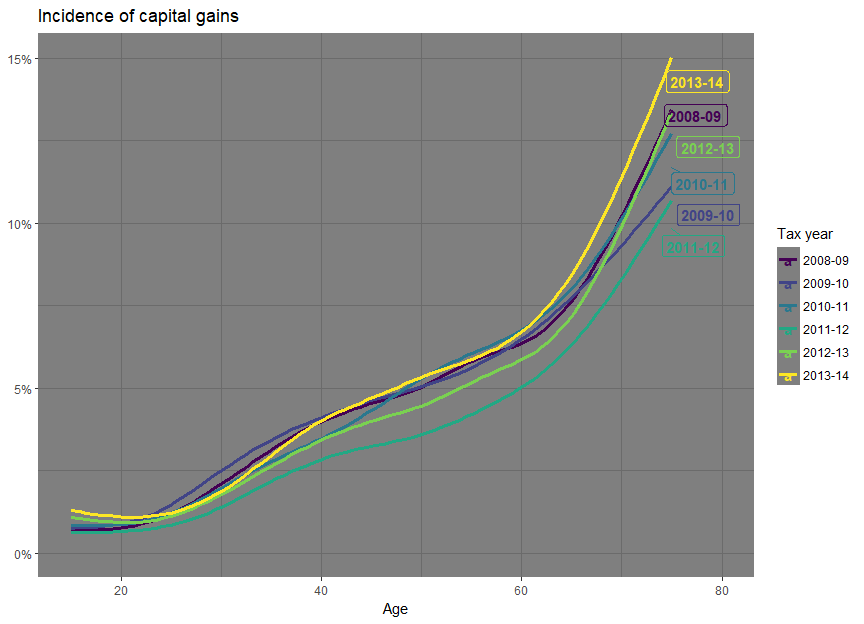set.seed(24841) sample_files_all %>% select(age_range, Net_CG_amt, fy.year) %>% merge(age_range_decoder, by = "age_range") %>% group_by(age_range_description) %>% mutate(min_age = ifelse(grepl("to", age_range_description), as.numeric(gsub("^([0-9]{2}).*$", "\\1", age_range_description)),
ifelse(grepl("70", age_range_description),
70,
15)),
max_age = min_age + 5,
age_imp = runif(n(), min_age, max_age)) %>%
select(-min_age, -max_age) %>%
filter(Net_CG_amt > 0) %>%
mutate(Age = round(age_imp)) %>%
group_by(fy.year, Age) %>%
summarise(mean_Net_CG = mean(Net_CG_amt),
sd_Net_CG = sd(Net_CG_amt)) %>%
ungroup %>%
mutate(last_fy = fy.year == max(fy.year) | fy.year == max(fy.year[fy.year != max(fy.year)])) %>%
group_by(fy.year) %>%
mutate(label = ifelse(Age == max(Age), fy.year, NA_character_),
label.y = mean(mean_Net_CG[Age > 70])) %>%
{
ggplot(., aes(x = Age, y = mean_Net_CG, color = factor(fy.year), group = factor(fy.year))) +
scale_y_continuous(label = dollar) +
scale_color_viridis(discrete = TRUE) +
geom_line(stat = "smooth", method = "auto", se = FALSE, size = 1.2) +
scale_alpha_discrete(range = c(0.5, 1)) +
geom_text(aes(label = label, y = label.y, size = if_else(last_fy %in% c("2012-13", "2013-14"), 2, 1),
nudge_x = if_else(last_fy, 1, 0)),
hjust = 0,
vjust = 0,
fontface = "bold",
na.rm = TRUE) +
scale_x_continuous(expand = c(0,0)) +
theme_dark() +
annotate("blank",
x = 85, y = 0) +
theme(axis.title.y = element_blank(),
plot.margin = unit(c(0,0,5,0), "pt"))
}
## Warning: Using alpha for a discrete variable is not advised.
## Warning: Ignoring unknown aesthetics: nudge_x
## Warning: Ignoring unknown aesthetics: x, y
## geom_smooth() using method = 'loess' and formula 'NULL'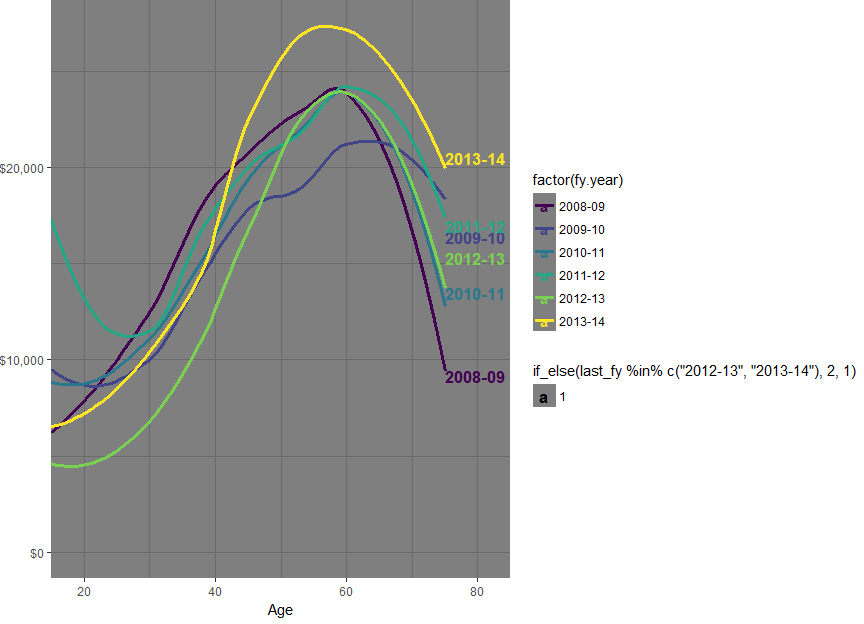sample_file %>%
mutate(Tot_inc_amt_noCG = Tot_inc_amt - Net_CG_amt,
Taxable_Income_noCG = pmaxC(Tot_inc_amt_noCG - Tot_ded_amt - NPP_loss_claimed - PP_loss_claimed, 0)) %>%
mutate(Taxable_Income_noCG_decile = ntile(Taxable_Income_noCG, 10)) %>%
filter(Taxable_Income_noCG_decile %in% c(1, 5, 10)) %>%
filter(Net_CG_amt > 0) %>%
rename(Age = age_imp) %>%
mutate(Taxable Income\n(excl CG) decile = factor(Taxable_Income_noCG_decile)) %>%
ggplot(aes(x = Age, fill = Taxable Income\n(excl CG) decile)) +
geom_density(size = 1.5, alpha = 0.7) +
scale_fill_viridis(discrete = TRUE) +
theme(legend.position = "right")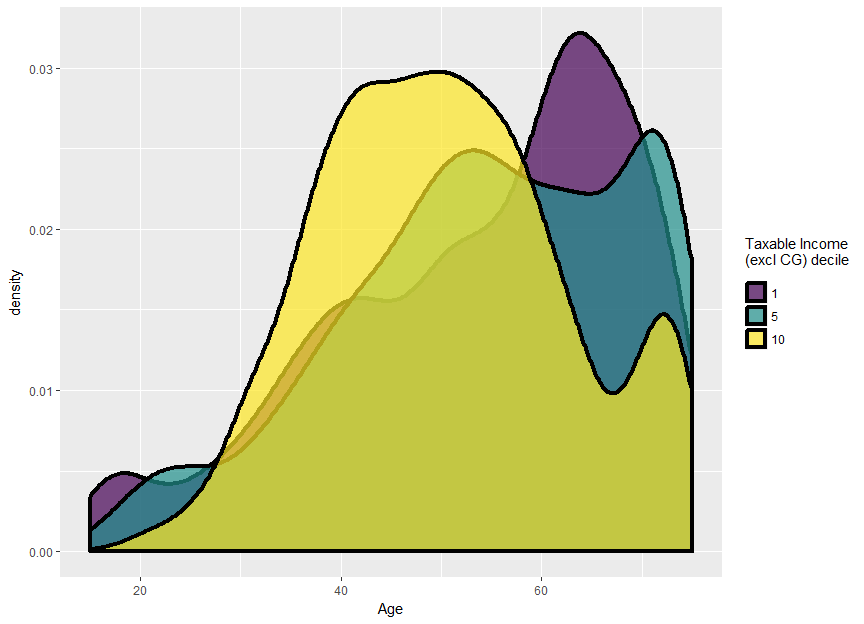if (FY.YEAR != "2013-14"){
stop("Check annotations in this chart before compiling")
}
sample_file %>%
filter(Net_CG_amt > 0, age_imp > 20) %>%
mutate(marginal_rate = income_tax(Taxable_Income + 1, fy.year = FY.YEAR) - income_tax(Taxable_Income, fy.year = FY.YEAR)) %>%
rename(Age = age_imp) %>%
ggplot(aes(x = Age, y = marginal_rate)) +
scale_y_continuous(label = percent) +
geom_smooth(aes(weight = 1), colour = viridis(2), size = 1.2) +
geom_smooth(aes(weight = Net_CG_amt), colour = viridis(2), size = 1.2) +
annotate("text",
x = c(57, 57),
y = c(0.335, 0.435),
label = c("Unweighted", "Weighted by CG amt"),
colour = viridis(2),
fontface = "bold",
hjust = 0) +
theme(axis.title.y = element_blank())
## geom_smooth() using method = 'gam' and formula 'y ~ s(x, bs = "cs")'
## geom_smooth() using method = 'gam' and formula 'y ~ s(x, bs = "cs")'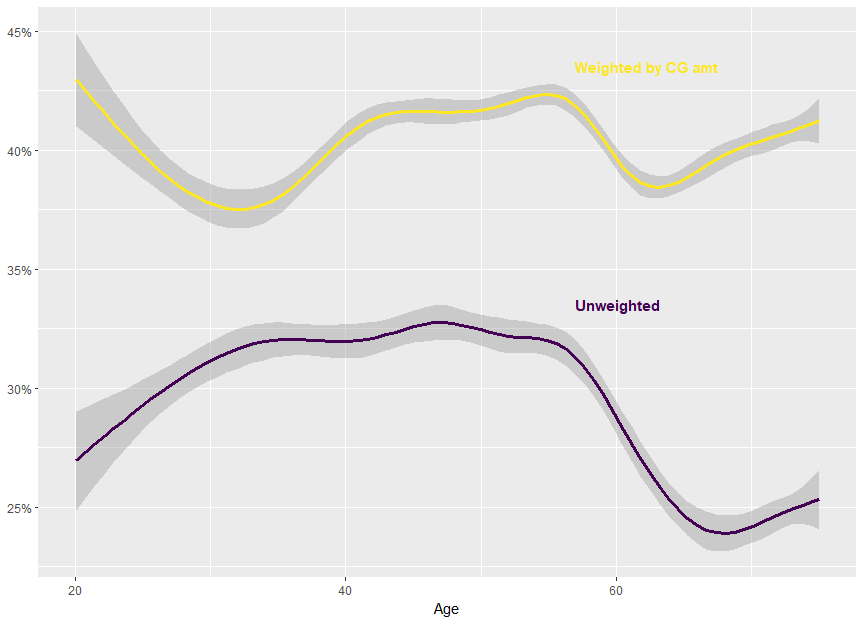if (FY.YEAR != "2013-14"){
stop("Check annotations in this chart before compiling")
}
sample_file %>%
filter(Net_CG_amt > 0, age_imp > 20) %>%
mutate(Taxable_Income_b4_CG = pmaxC(Taxable_Income - Net_CG_amt, 0),
marginal_rate_b4_CG = income_tax(Taxable_Income_b4_CG + 1, fy.year = FY.YEAR) - income_tax(Taxable_Income_b4_CG, fy.year = FY.YEAR)) %>%
rename(Age = age_imp) %>%
ggplot(aes(x = Age, y = marginal_rate_b4_CG)) +
scale_y_continuous(label = percent) +
geom_smooth(aes(weight = 1),  colour = viridis(2), size = 1.2) +
geom_smooth(aes(weight = Net_CG_amt), colour = viridis(2), size = 1.2) +
annotate("text",
x = c(31, 35),
y = c(0.315, 0.225),
label = c("Unweighted", "Weighted by CG amt"),
colour = viridis(2),
fontface = "bold",
hjust = 0) +
theme(axis.title.y = element_blank())
## geom_smooth() using method = 'gam' and formula 'y ~ s(x, bs = "cs")'
## geom_smooth() using method = 'gam' and formula 'y ~ s(x, bs = "cs")'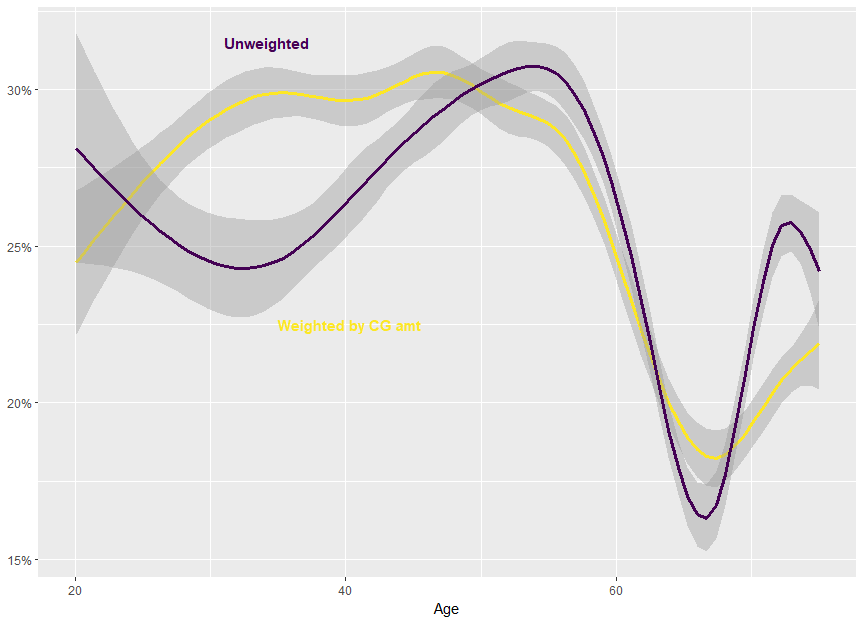n_prop_invstrs <-
sample_file %$% sum((Gross_rent_amt > 0) * WEIGHT) n_NGs <- sample_file %$%
sum((Net_rent_amt < 0) * WEIGHT)

val_NG_losses <-
sample_file %$% sum(abs(pminC(Net_rent_amt, 0) * WEIGHT)) NG_tax_exp <- sample_file %>% mutate(tax = income_tax(Taxable_Income, fy.year = FY.YEAR), new_tax = income_tax(Taxable_Income - pminC(Net_rent_amt, 0), fy.year = FY.YEAR), diff = new_tax - tax) %$%
sum(diff * WEIGHT)

There were 2~million property investors. Of these, 1.25~million were negative gearing. Losses claimed totaled $10.7~billion. This delivered a tax expenditure (by revenue foregone) of$3.63~billion.

sample_file %>%
filter(between(Sw_amt, 0, 250e3)) %>%
rename(Salary = Sw_amt) %>%
ggplot(aes(x = Salary, y = as.numeric(Net_rent_amt < 0))) +
geom_smooth(colour = viridis(2), size = 1.5) +
scale_y_continuous(label = percent) +
scale_x_continuous(label = dollar) +
theme(axis.title.y = element_blank())
## geom_smooth() using method = 'gam' and formula 'y ~ s(x, bs = "cs")'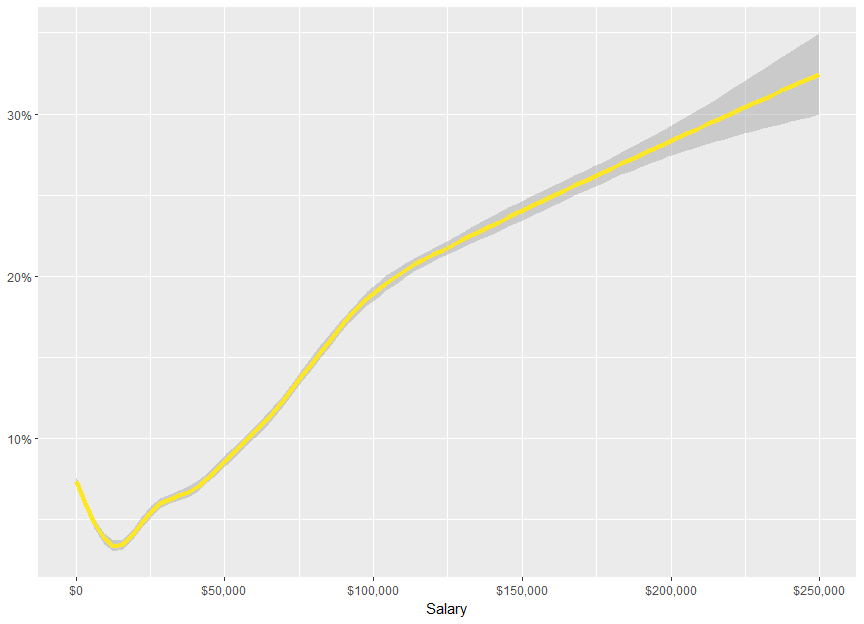NG_by_taxBracket <-
sample_file %>%
mutate(Tax_bracket = cut(Taxable_Income,
breaks = c(-Inf, 18200, 37e3, 80e3, 180e3, Inf),
labels = c("$0-$18,200", "$18,201-$37,000",
"$37,001-$80,000", "$80,001-$180,000",
"Over 180,000"), ordered_results = TRUE, include.lowest = TRUE)) %>% group_by(Tax_bracket) %>% summarise(n_NG = wsum(Net_rent_amt < 0, WEIGHT), n = sum(WEIGHT)) %>% arrange(Tax_bracket) NG_by_taxBracket %>% mutate(Number negative gearing = comma(n_NG), \\% = percent(n_NG / n)) %>% select(Tax bracket = Tax_bracket, Number negative gearing, \\%) %>% kable(align = "rrr")  Tax bracket Number negative gearing %0-$18,200 161,800 6.4%$18,201-$37,000 180,950 5.8%$37,001-$80,000 469,500 9.8%$80,001-$180,000 350,800 16.4% Over$180,000 85,200 22.4%
NG_by_taxBracket_tax_benefit <-
sample_file %>%
mutate(Tot_inc_amt_NoNG = Tot_inc_amt - Net_rent_amt + pmaxC(Net_rent_amt, 0),
Taxable_Income_noNG = pmaxC(Tot_inc_amt_NoNG - Tot_ded_amt - NPP_loss_claimed - PP_loss_claimed, 0),
tax_current = income_tax(Taxable_Income, fy.year = FY.YEAR),
tax_noNG = income_tax(Taxable_Income_noNG, fy.year = FY.YEAR),
change = tax_noNG - tax_current) %>%
mutate(Tax_bracket = cut(Taxable_Income,
breaks = c(-Inf, 18200, 37e3, 80e3, 180e3, Inf),
labels = c("$0-$18,200",
"$18,201-$37,000",
"$37,001-$80,000",
"$80,001-$180,000",
"Over 180,000"), ordered_results = TRUE, include.lowest = TRUE)) %>% group_by(Tax_bracket) %>% summarise(total_tax_change = sum(change * WEIGHT), avg_tax_change = mean(change)) %>% arrange(Tax_bracket) NG_by_taxBracket_tax_benefit %>% mutate(Total tax change = grattan_dollar(total_tax_change), Average tax change = grattan_dollar(avg_tax_change)) %>% select(Tax bracket = Tax_bracket, Total tax change, Average tax change) %>% kable(align = paste0(rep("r", ncol(.)), collapse = "")) Tax bracket Total tax change Average tax change0-$18,200$97,261,913 $39$18,201-$37,000$333,873,994 $108$37,001-$80,000$1,246,976,877 $259$80,001-$180,000$1,288,682,051 $603 Over$180,000 $648,280,796$1,706
NG_by_taxable_income_decile <-
sample_file %>%
mutate(Taxable_Income_decile = ntile(Taxable_Income, 10)) %>%
group_by(Taxable_Income_decile) %>%
summarise(n_NG = wsum(Net_rent_amt < 0, WEIGHT),
n = sum(WEIGHT)) %>%
arrange(Taxable_Income_decile) 
NG_by_taxable_income_decile %>%
mutate(Number negative gearing = comma(n_NG),
\\% = percent(n_NG / n)) %>%
mutate(Taxable Income decile = factor(Taxable_Income_decile)) %>%
select(Taxable Income decile,
Number negative gearing,
\\%) %>%
kable(align = "rrrr") 
Taxable Income decile Number negative gearing %
1 109,550 8.5%
2 55,750 4.3%
3 64,950 5.0%
4 82,400 6.4%
5 93,700 7.2%
6 106,600 8.2%
7 130,750 10.1%
8 157,950 12.2%
9 192,600 14.9%
10 254,000 19.6%
NG_tax_benefit_taxable_income_decile <-
sample_file %>%
mutate(Tot_inc_amt_NoNG = Tot_inc_amt - Net_rent_amt + pmaxC(Net_rent_amt, 0),
Taxable_Income_noNG = pmaxC(Tot_inc_amt_NoNG - Tot_ded_amt - NPP_loss_claimed - PP_loss_claimed, 0),
tax_current = income_tax(Taxable_Income, fy.year = FY.YEAR),
tax_noNG = income_tax(Taxable_Income_noNG, fy.year = FY.YEAR),
change = tax_noNG - tax_current) %>%
mutate(Taxable_Income_decile = ntile(Taxable_Income, 10)) %>%
group_by(Taxable_Income_decile) %>%
summarise(tax_diff = sum(change * WEIGHT)) %>%
ungroup %>%
mutate(tax_diff_prop = tax_diff / sum(tax_diff)) %>%
arrange(Taxable_Income_decile) %>%
mutate(decile_by = "Taxable income")

NG_tax_benefit_taxable_income_decile_noNG <-
sample_file %>%
mutate(Tot_inc_amt_NoNG = Tot_inc_amt - Net_rent_amt + pmaxC(Net_rent_amt, 0),
Taxable_Income_noNG = pmaxC(Tot_inc_amt_NoNG - Tot_ded_amt - NPP_loss_claimed - PP_loss_claimed, 0),
tax_current = income_tax(Taxable_Income, fy.year = FY.YEAR),
tax_noNG = income_tax(Taxable_Income_noNG, fy.year = FY.YEAR),
change = tax_noNG - tax_current) %>%
mutate(Taxable_Income_decile = ntile(Taxable_Income_noNG, 10)) %>%
group_by(Taxable_Income_decile) %>%
summarise(tax_diff = sum(change * WEIGHT)) %>%
ungroup %>%
mutate(tax_diff_prop = tax_diff / sum(tax_diff)) %>%
arrange(Taxable_Income_decile) %>%
mutate(decile_by = "Taxable income before NG")

bind_rows("Current" = NG_tax_benefit_taxable_income_decile,
"Before NG" = NG_tax_benefit_taxable_income_decile_noNG) %>%
mutate(Taxable income decile = factor(Taxable_Income_decile)) %>%
ggplot(aes(x = Taxable income decile, y = tax_diff_prop, fill = decile_by)) +
geom_bar(stat = "identity") +
facet_grid(~decile_by) +
scale_y_continuous(label = percent,
expand = c(0,0),
limits = c(0, round(max(c(NG_tax_benefit_taxable_income_decile_noNG$tax_diff_prop, NG_tax_benefit_taxable_income_decile$tax_diff_prop)), 1)))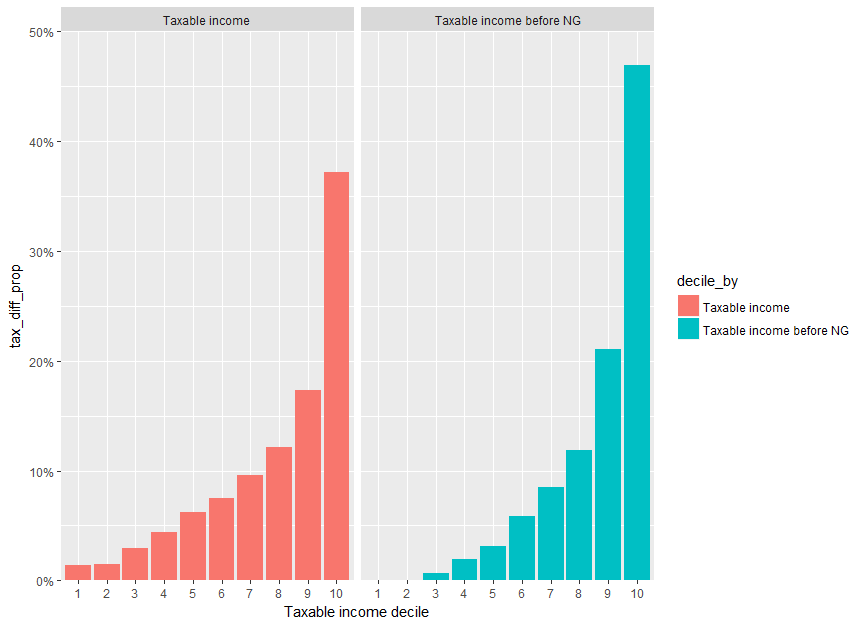NG_tax_benefit_taxable_income_decile_prev <-
sample_file_prev %>%
mutate(Tot_inc_amt_NoNG = Tot_inc_amt - Net_rent_amt + pmaxC(Net_rent_amt, 0),
Taxable_Income_noNG = pmaxC(Tot_inc_amt_NoNG - Tot_ded_amt - NPP_loss_claimed - PP_loss_claimed, 0),
tax_current = income_tax(Taxable_Income, fy.year = FY.YEAR),
tax_noNG = income_tax(Taxable_Income_noNG, fy.year = FY.YEAR),
change = tax_noNG - tax_current) %>%
mutate(Taxable_Income_decile = ntile(Taxable_Income, 10)) %>%
group_by(Taxable_Income_decile) %>%
summarise(tax_diff = sum(change * WEIGHT)) %>%
ungroup %>%
mutate(tax_diff_prop = tax_diff / sum(tax_diff)) %>%
arrange(Taxable_Income_decile) %>%
mutate(decile_by = "Taxable income")

NG_tax_benefit_taxable_income_decile_noNG_prev <-
sample_file_prev %>%
mutate(Tot_inc_amt_NoNG = Tot_inc_amt - Net_rent_amt + pmaxC(Net_rent_amt, 0),
Taxable_Income_noNG = pmaxC(Tot_inc_amt_NoNG - Tot_ded_amt - NPP_loss_claimed - PP_loss_claimed, 0),
tax_current = income_tax(Taxable_Income, fy.year = FY.YEAR),
tax_noNG = income_tax(Taxable_Income_noNG, fy.year = FY.YEAR),
change = tax_noNG - tax_current) %>%
mutate(Taxable_Income_decile = ntile(Taxable_Income, 10)) %>%
group_by(Taxable_Income_decile) %>%  summarise(tax_diff = sum(change * WEIGHT)) %>%
ungroup %>%
mutate(tax_diff_prop = tax_diff / sum(tax_diff)) %>%
arrange(Taxable_Income_decile) %>%
mutate(decile_by = "Taxable income before NG")

bind_rows("Current" = NG_tax_benefit_taxable_income_decile,
"Before NG" = NG_tax_benefit_taxable_income_decile_noNG,
"Current (prev fy)" = NG_tax_benefit_taxable_income_decile_prev,
"Before NG (prev fy)" = NG_tax_benefit_taxable_income_decile_noNG_prev,
.id = "df_id") %>%
mutate(Taxable income decile = factor(Taxable_Income_decile)) %>%
mutate(financial_year = ifelse(grepl("prev fy", df_id), PREV.FY.YEAR, FY.YEAR)) %>%
ggplot(aes(x = Taxable income decile, y = tax_diff_prop, fill = financial_year)) +
geom_bar(stat = "identity", position = "dodge") +
scale_fill_viridis(discrete = TRUE, begin = 0, end = 0.3333) +
facet_grid(~decile_by) +
scale_y_continuous(label = percent,
expand = c(0,0),
limits = c(0, round(max(c(NG_tax_benefit_taxable_income_decile_noNG$tax_diff_prop, NG_tax_benefit_taxable_income_decile$tax_diff_prop)), 1))) +
theme(legend.margin = unit(0, "lines"),
legend.title = element_blank(),
legend.position = c(0.00, 1.025),
legend.background = element_blank(),

legend.justification = c(0, 1),
axis.title.y = element_blank(),
strip.background = element_rect(color = grey(0.8), fill = grey(0.8)),
strip.text = element_text(colour = "white", face = "bold"))
## Warning: legend.margin must be specified using margin(). For the old
## behavior use legend.spacing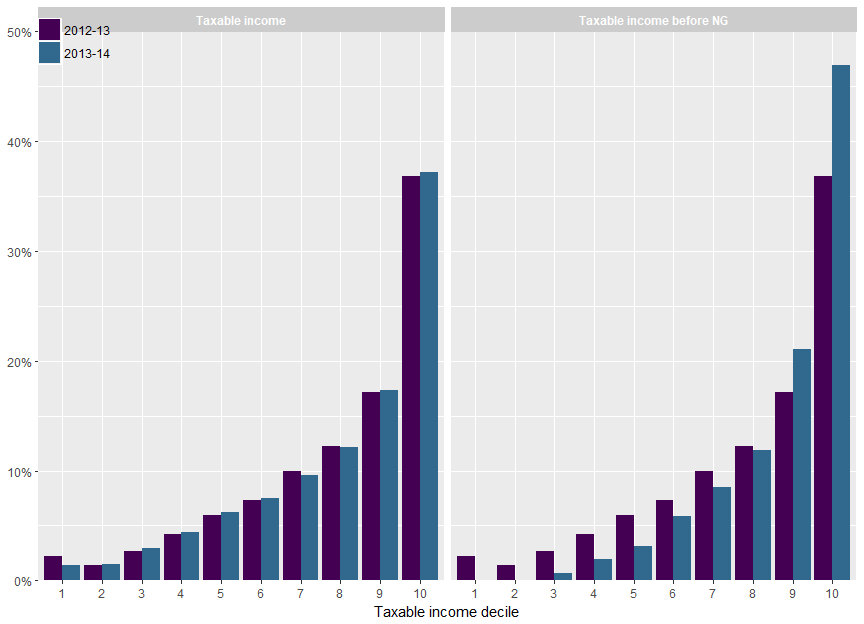p <-
ggplot(NULL) +
geom_smooth(data = sample_file,
aes(x = age_imp, y = as.numeric(Net_rent_amt < 0)),
colour = viridis(2),
size = 1.2) +
geom_smooth(data = filter(sample_file, Gross_rent_amt > 0),
aes(x = age_imp,
y = as.numeric(Net_rent_amt < 0)),
colour = viridis(2),
size = 1.2) +
scale_y_continuous(label = percent) +
xlab("Age") +
coord_cartesian(ylim = c(0,1)) +
theme(axis.title.y = element_blank())

if (FY.YEAR == "2013-14"){
p <-
p +
annotate("text",
x = c(38, 38),
y = c(0.18, y = 0.80),
label = c("All taxpayers", "Property investors"),
hjust = c(0.5, 0),
colour = viridis(2),
fontface = "bold")
} else {
p <- p +
theme(legend.position = "right")
}

p
## geom_smooth() using method = 'gam' and formula 'y ~ s(x, bs = "cs")'
## geom_smooth() using method = 'gam' and formula 'y ~ s(x, bs = "cs")'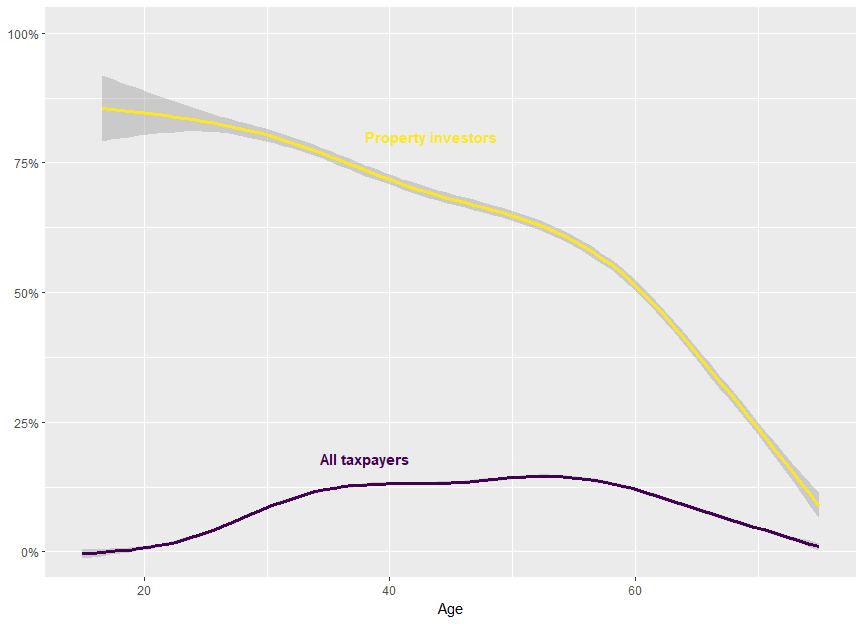age_res = 1
inc_res = 10000

sample_file %>%
mutate(Tot_inc_amt_NoNG = Tot_inc_amt - Net_rent_amt + pmaxC(Net_rent_amt, 0),
Taxable_Income_noNG = pmaxC(Tot_inc_amt_NoNG - Tot_ded_amt - NPP_loss_claimed - PP_loss_claimed, 0),
tax_current = income_tax(Taxable_Income, fy.year = FY.YEAR),
tax_noNG = income_tax(Taxable_Income_noNG, fy.year = FY.YEAR),
change = tax_noNG - tax_current) %>%

# This excludes income losses (barely any anyway)
# and high income earners
filter(between(Tot_inc_amt_NoNG,
0,
upper_ylim <<- quantile(.$Tot_inc_amt_NoNG[.$Tot_inc_amt_NoNG > 0], probs = 0.95))) %>%

mutate(Age = age_res * round(age_imp / age_res),
Total Income (before NG) = inc_res * round(Tot_inc_amt_NoNG / inc_res)) %>%
group_by(Age, Total Income (before NG)) %>%
summarise(n_NG = sum((Net_rent_amt < 0) * WEIGHT),
prop_NG = mean(Net_rent_amt < 0),
tot_tax_benefit = sum(change * WEIGHT),
avg_tax_benefit = mean(change)) %>% ungroup %>%

ggplot(aes(x = Age, y = Total Income (before NG), fill = prop_NG)) +
geom_bin2d(stat = "identity") +
scale_fill_viridis("% NG", labels = percent) +
scale_y_continuous(expand = c(0,0), label = grattan_dollar) +
scale_x_continuous(expand = c(0,0)) +
theme_dark() +
theme(legend.title = element_blank(),
plot.margin = unit(c(0,0,0,0), "pt"))# %>%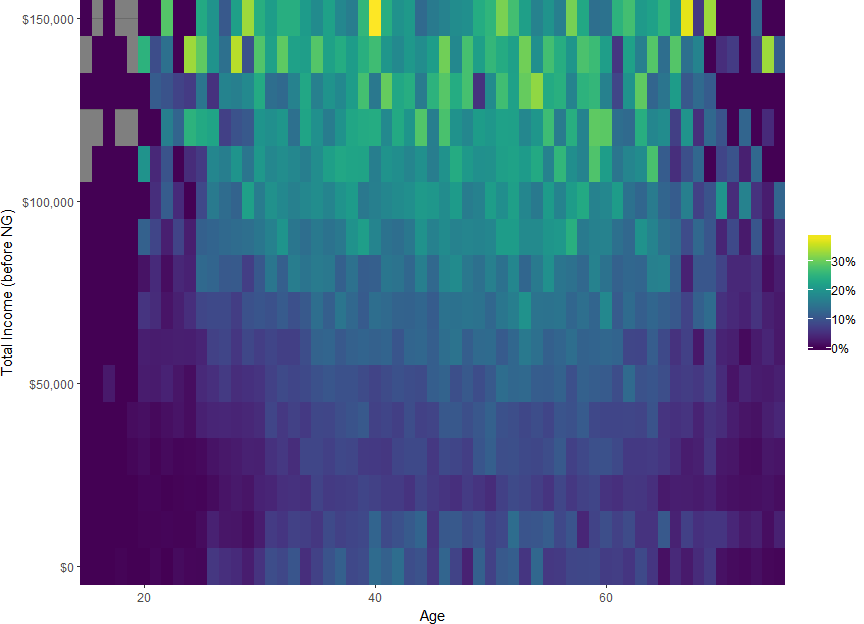#align_baptiste(.)
age_res = 1
inc_res = 10000

sample_file %>%
mutate(Tot_inc_amt_NoNG = Tot_inc_amt - Net_rent_amt + pmaxC(Net_rent_amt, 0),
Taxable_Income_noNG = pmaxC(Tot_inc_amt_NoNG - Tot_ded_amt - NPP_loss_claimed - PP_loss_claimed, 0),
tax_current = income_tax(Taxable_Income, fy.year = FY.YEAR),
tax_noNG = income_tax(Taxable_Income_noNG, fy.year = FY.YEAR),
change = tax_noNG - tax_current) %>%

# This excludes income losses (barely any anyway)
# and high income earners
filter(between(Tot_inc_amt_NoNG,
0,
upper_ylim <<- quantile(.$Tot_inc_amt_NoNG[.$Tot_inc_amt_NoNG > 0], probs = 0.95))) %>%

mutate(Age = age_res * round(age_imp / age_res),
Total Income (before NG) = inc_res * round(Tot_inc_amt_NoNG / inc_res)) %>%
group_by(Age, Total Income (before NG)) %>%
summarise(n_NG = sum((Net_rent_amt < 0) * WEIGHT),
prop_NG = mean(Net_rent_amt < 0),
tot_tax_benefit = sum(change * WEIGHT),
avg_tax_benefit = mean(change)) %>% ungroup %>%

ggplot(aes(x = Age, y = Total Income (before NG), fill = avg_tax_benefit)) +
geom_bin2d(stat = "identity") +
scale_fill_viridis("Tax benefit", labels = grattan_dollar) +
scale_y_continuous(expand = c(0,0), label = grattan_dollar) +
scale_x_continuous(expand = c(0,0)) +
theme_dark() +
theme(legend.title = element_blank())